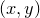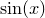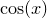# 10.2 Multiplot mode

Pyxplot has two modes in which it can produce graphical output. In singleplot mode, the default, each time the plot command is issued, the canvas is wiped clean and the new plot is placed alone on a blank page. In multiplot mode, vector graphics objects accumulate on the canvas. Each time the plot command is issued, the new graph is placed on top of any other objects which were already on the canvas, and many plots can be placed side-by-side.

The user can switch between these two modes of operation by issuing the commands set multiplot and set nomultiplot. The set origin command is required for multiplot mode to be useful when placing plots side-by-side: it sets the position on the page of the lower-left corner of the next plot. It takes a comma-separatedcoordinate pair, which may have units of length, or, if dimensionless, are assumed to be measured in centimetres. The following example plots a graph ofto the left of a plot of:

set multiplot
set width 8
plot sin(x)
set origin 10,0
plot cos(x)